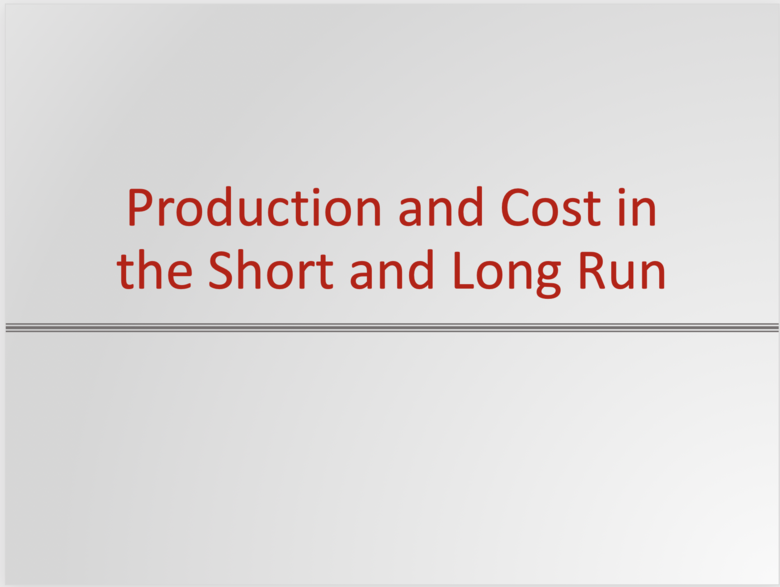Subject:
Economics
Material Type:
Module
Level:
Community College / Lower Division, College / Upper Division
Provider:
Ohio Open Ed Collaborative
Tags:
Accounting and Economic Profit, Explicit and Implicit Costs, Fixed and Variable Costs, Fixed and Variable Inputs, Long and Short Run, Oss0042
Language:
English
Media Formats:
Text/HTML

# Production and Cost in the Short and Long Run Resources## Overview

This topic covers the analysis of a firm’s costs in the short and long run.  It explains the important difference between accounting and economic costs (and how that affects the definition of accounting and economic profits).  It also makes the distinction between fixed and variable costs.

# Learning Objectives

1. Define explicit and implicit costs (8,9)
2. Determine accounting and economic profit (9)
3. Evaluate production decisions in the short run (8)
4. Define fixed and variable inputs (8)
5. Determine costs in the short run (9)
6. Evaluate production decisions in the long run (8)
7. Describe costs in the long run (9)

NOTE: This Module meets Ohio TAG's 8 and 9 for an Intro to Microeconomics Course OSS004

# Recommended Textbook Resources

## Principles of Microeconomics 2eChapter 7. Introduction to Production, Costs, and Industry Structure

Chapter 7.6. Key Terms

Chapter 7.7. Key Concepts and Summary

Chapter 7.8. Self-Check Questions

Chapter 7.9. Review Questions

Chapter 7.10. Critical Thinking Questions

Chapter 7.11. Problems

# Supplemental Content/Alternative Resources

## Khan Academy AP Microeconomics: Production, Cost and the Perfect Competition Model

This is a video presentation of the material similar to that in the text, plus it includes an introduction to perfect competition. The recommended text does a good job presenting the theory so this video is simply a different approach that some students might find beneficial.

# Active Learning Exercise

 Game: #3 Course: Micro Level: Principles and up Subject(s): Short-run production/production costs Objective: To help students understand TPP, APP, MPP, TFC, TVC, TC, MC, AFC, AVC, AC (and the associated short-run production and cost curves) Reference and contact: Neral, John. "Widget Production in the Classroom." Classroom Expernomics, 2(1), Spring 1993, pp. 7-8. or contact Dr. John Neral; Department of Economics; Frostburg State University; Frostburg, MD 21532; ph.: 1-301-689-4265 j_neral@fre.fsu.umd.edu Abstract: Provide students with (or ask them to bring) lots of paper and a (working) stapler. Divide the class into large groups (say, eight or more per group). Provide each group with half a table of production room (the shop floor). That plus the stapler is the fixed capital input (K=1). The group is to produce as many widgets as possible (fold the paper twice and staple it) within one workday, say, a 30-second time period. Start with no labor (L=0) to produce Q=0, never minding the stares. Then increase to L=1; have the group record total production (TPP) in the 30-second time-span. Then increase to L=2 and so on until diminishing returns set in (perhaps even negative returns if you like). Let the groups compute, tabulate, and graph their TPP, APP, and MPP. Then assign costs, e.g., K=$10, L=$5, and let the groups compute, tabulate, and graph the cost variables. They should get something more or less bizarrely similar to the nice textbook curves. (They'll learn about the convenience of abstraction pretty quickly this way.) Class size: Small and up. For very large classes, part of the class could watch with amusement; they'll get the point. Alternatively, part of the class could do the production runs; another part of the class to computation, tabulation, and graphing. Time: One class period Variations: None indicated

# Questions and Problems

Instructors can add a Google Doc of the Production and Cost in the Long and Short Run Questions and Problems to their Google Drive or download a Word File of the Production and Cost in the Short and Long Run Questions and Problems.

## Questions

1. Complete the following table:
 Output Total Cost Variable Cost Fixed Cost ATC AVC AFC MC 0 100 * * * * 1 110 10 2 12 3 137 4 20 5 87

### Use the graph below to answer questions 1 - 41. At what quantity is average variable cost minimized?

2. At what quantity is average total cost minimized?

3. If average variable cost is $5 when output is 20 units, what is this firm’s fixed cost? 4. What is AFC at the shut-down point? ### Use Figure 2 to answer the following questions:1. At what output levels are there the following 1. Decreasing returns to scale? 2. Constant returns to scale? 3. Increasing returns to scale? 2. Over time, what range of output would you expect firms to produce, assuming any level of output is feasible? ## Answers: 1.  Output Total Cost Variable Cost Fixed Cost ATC AVC AFC MC 0 100 0 100 * * * * 1 110 10 100 110 10 100 10 2 122 22 100 61 11 50 12 3 137 37 100 45.67 12.33 33.33 15 4 157 57 100 39.25 14.25 25 20 5 187 87 100 37.40 17.40 20 30 1. 15 2. 20 3.$40  (ATC – AVC = AFC so $7 -$5 = $2; FC = AFC*q so$2 * 20 = $40) 4.$2.57  (Shutdown at q = 15; $40/15 =$2.67)
5.
1. Q > 3,000
2. 1,000 < Q < 3,000
3. Q < 1,000
4. Between 1,000 and 3,000; any other level of output would put the firm at a cost disadvantage.